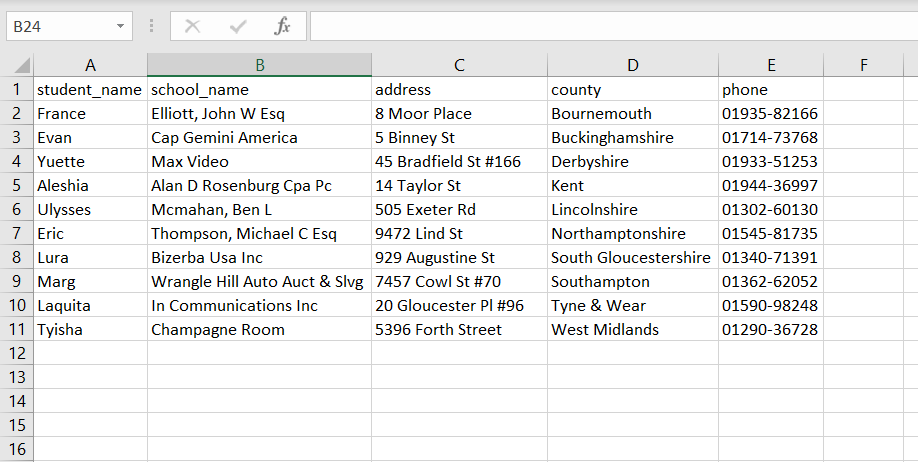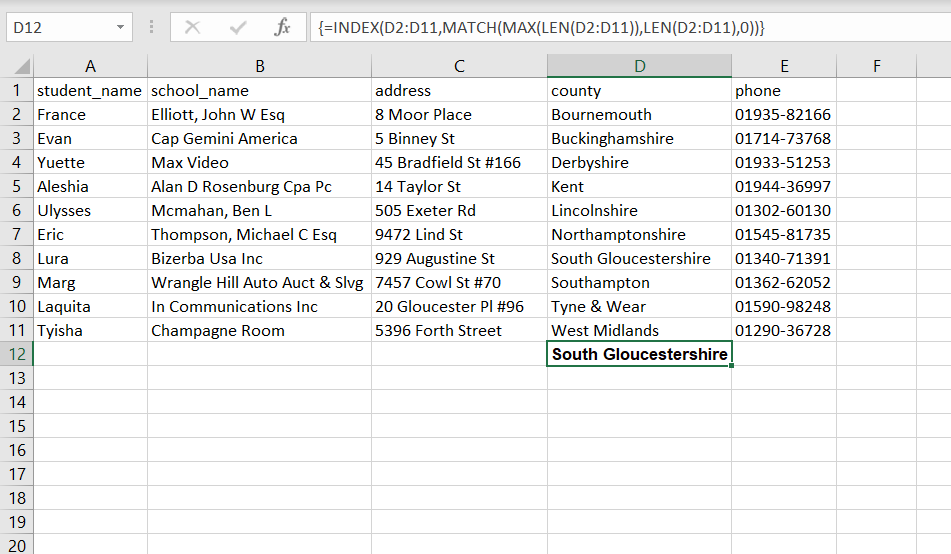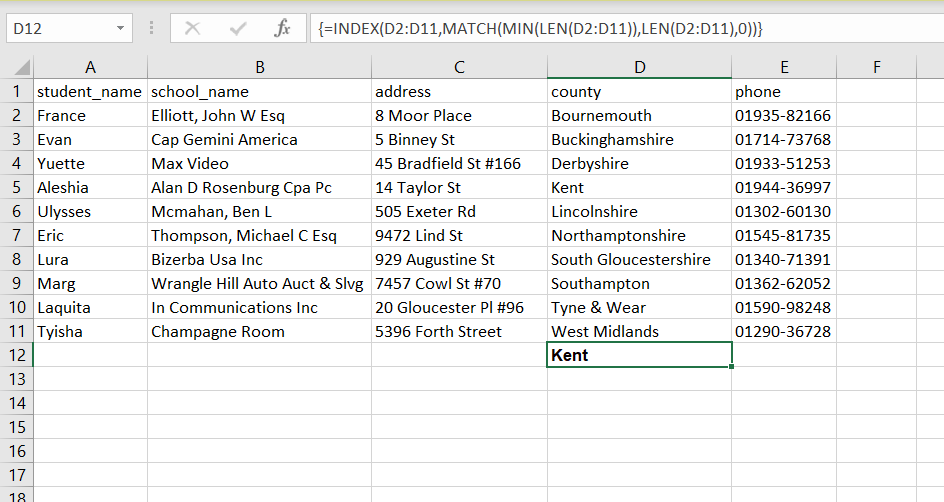Open in App
Not now

# How to Find the Longest or Shortest Text String in a Column in Excel?

• Last Updated : 06 Oct, 2022

In this article, we will see how to find the longest or shortest text string in a column in Excel? Usually, for finding the longest or shortest string we can visit the all string in columns one by one and compare them to get results. This seems to work when you have less amount of data in an excel sheet. This is not a particle with a large dataset in Excel. Let’s see how you can find the longest or shortest string in the column by using just one single line of formula in Excel.

## Longest Text String in a Column:

We will use the below formula to get the longest text string in a column in an excel file.

Syntax:

`=INDEX(range,MATCH(MAX(LEN(range)),LEN(range),0)`

Parameters:

• INDEX: Returns the value of the given position in the column
• MATCH: Locate the position of a lookup value in a column
• MAX: Returns the largest value
• LEN: Returns the length of the given string
• range: Input range of column

### Explanation of Formula:

Let’s start with the inner parenthesis value. LEN(range) will return the array of the length of the cell that comes under the input range. Now MAX will return the maximum numeric value it that is the maximum length from the given array. Now this two are passed to MATCH. It will return the position of a lookup value in a column. MATCH is used to perform an exact match. Now INDEX will return the value of the input position of columns.

Example:

Let’s suppose we have the below excel file with 5 columns and we want to find the longest text string in a column ‘country’ that is column D. We will use the above formula to find it.Now, let’s see how to define the formula for this and what will be the output of it.Here, we first select the blank cell in column D. Then we enter a formula in that cell and you can see the output of it in the above image. In the above image, you can see we select range from D2 to D11. This is the range of the column in which we want to find the longest text string.

Note: After entering the formula pressing enter will not give the result, you must press Shift + Ctrl + Enter keys together. This will return the longest text string that has been extracted in the column.

## Shortest Text String in a Column:

We will use the below formula to get the Shortest text string in a column in an excel file.

Syntax:

`=INDEX(range,MATCH(MIN(LEN(range)),LEN(range),0)`

Parameters:

• INDEX: Returns the value of the given position in the column
• MATCH: Locate the position of a lookup value in a column
• MIN: Returns the shortest value
• LEN: Returns the length of the given string
• range: Input range of column

### Explanation of Formula:

Let’s start with the inner parenthesis value. LEN(range) will return the array of the length of the cell that comes under the input range. Now MIN will return the minimum numeric value of it that is the minimum length from the given array. Now this two are passed to MATCH. It will return the position of a lookup value in a column. MATCH is used to perform an exact match. Now INDEX will return the value of the input position of columns.

Example:

Let’s suppose we have the below excel file with 5 columns and we want to find the shortest text string in a column ‘country’ that is column D. We will use the above formula to find it.Now, let’s see how to define the formula for this and what will be the output of it.Here, we first select the blank cell in column D. Then we enter a formula in that cell and you can see the output of it in the above image. In the above image, you can see we select range from D2 to D11. This is the range of the column in which we want to find the shortest text string. Here also remember that after entering the formula pressing enter will not give the result, you must press Shift + Ctrl + Enter keys together.

My Personal Notes arrow_drop_up